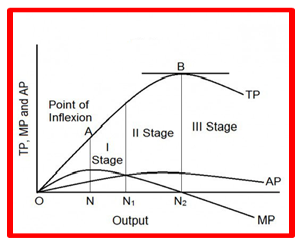## Pricing Table Particle

• Basic
• Standard Compliant Channels
• $50 • Completely synergize resource taxing relationships via premier market • 1 GB of space • Support at$25/hour
• Standard Compliant Channels
• $100 • Completely synergize resource taxing relationships via premier market • 10 GB of space • Support at$15/hour
• Platinum
• Standard Compliant Channels
• $250 • Completely synergize resource taxing relationships via premier market • 30 GB of space • Support at$5/hour

### Search Our Site

Theory of Production
Microeconomics
Topic Nine

# Production and Productivity

Production is simply the conversion of inputs into outputs.

### The Short Run versus the Long Run in Production Decisions

The short run is defined as the time horizon over which the scale of operation is fixed and the only variable input is labour; therefore, at least one input is fixed. In this period, the firm can make decisions to increase some of factors of production but not all.  By contrast, the long run is defined as a period in which all inputs are variable.  In this case, all of the factors of production are variable because the firm will have sufficient time to adjust its inputs.

### Measures of Output: Total, Average, and Marginal Product

There are three main measures of output which are total product, average product and marginal product.  Total product (TP) is the total amount of output produced. The average product (AP) is the total product divided by the number of units of the variable input employed. It is the output of each unit of input

Marginal product (MP) is the increase in output resulting from a one-unit increase in the amount of the variable input employed.

# The Relationship between the Product Curves

When the marginal product of labour curve rises, the firm experiences increasing marginal returns.  That is, the marginal product of an additional worker exceeds the marginal product of the previous worker. At this time, the rate of increase in total product is accelerating. When the marginal product of labour curve falls, the firm experiences diminishing marginal returns.  That is, the marginal product of an additional worker falls short of the marginal product of the previous worker. This is when the total product grows at a diminishing rate. As the marginal product continues to decrease, it will eventually become zero, then negative. This is when the total product declines.  The law of diminishing returns states that as successive units of a variable resource are added to a fixed resource, the marginal product of the variable input eventually diminishes, assuming all units of variable inputs, workers in this case, are of equal quality.

### Stages of Production

The production process has three stages.

### Stage One

Stage one is the period of most growth in a company's production. In this period, each additional variable input will produce more products. All three curves, the total product curve, the average product curve and the marginal product curve, are increasing and positive in this stage.

### Stage Two

Stage two is the period where marginal returns start to decrease. Each additional variable input will still produce additional units but at a decreasing rate. This is because the law of diminishing returns starts to set in. The total product curve is still rising in this stage, while the average and marginal curves both start to decline.

### Stage Three

In stage three, marginal returns start to become negative. Adding more variable inputs to the fixed amount of fixed inputs becomes counterproductive.  In this stage, the total product curve starts to trend down, the average product curve continues its descent and the marginal curve becomes negative.